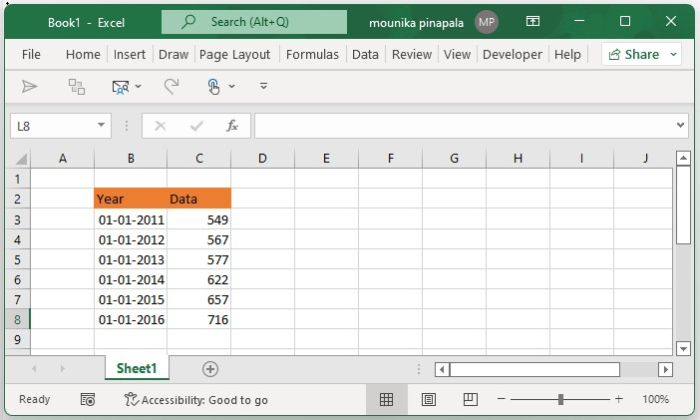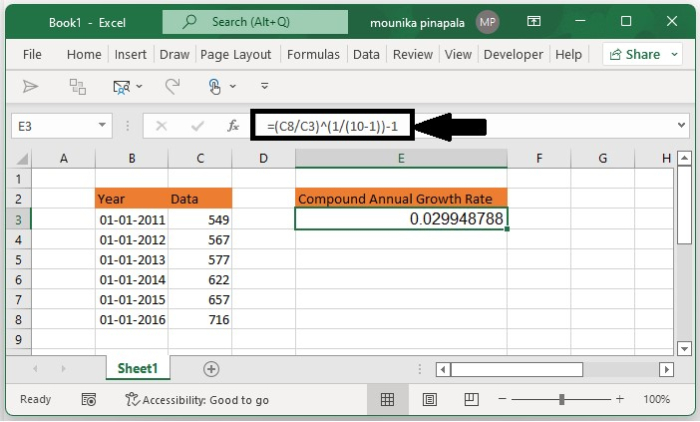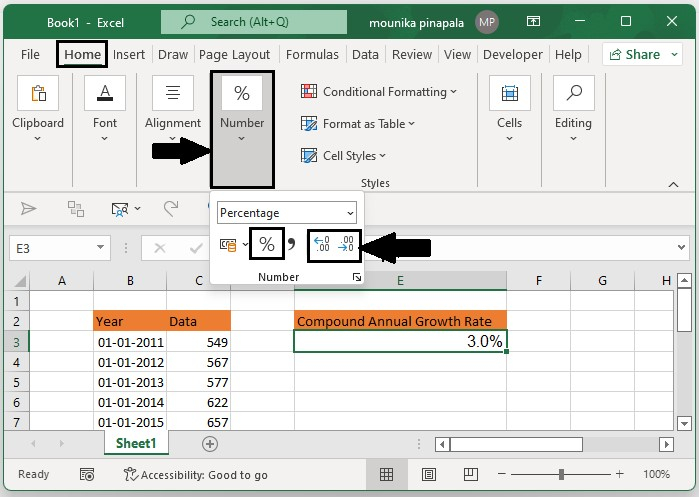# How to calculate average/compound annual growth rate in the Excel?

For you to calculate the compound annual growth rate (CAGR) in an Excel sheet, there is a basic formula that you need to follow =(End Value/Start Value)^(1/Periods)-1 you can easily apply this formula for this kindly follow the below steps −

## Step 1

Open a Microsoft excel sheet and enter the data as shown in below. You can change the data as per your requirement. Kindly find the below screenshot for your reference.## Step 2

Now you need to select a blank cell and enter the below given formula into the blank cell and press the enter key so that you will get the compound annual growth rate as shown in the below screenshot for your reference.

=(C8/C3)^(1/(10-1))-1Note − In the above given formula, C8 is the cell with the end value, C3 is the cell with the starting value, and 10-1 is the period that you have given between the start value and end value. You can change this as per your need.

## Step 3

At times the calculation result will not be in percentage format, for this, you need to select the result cell and then click on the percentage % icon under the number on the home tab to change the number obtained to the percentage format. And then change its decimal places by using the increase decimal button or decrease decimal button as shown in the below screenshot for your reference.## Conclusion

In this tutorial, we used a simple example to demonstrate how you can calculate the average or compound annual growth rate in an Excel sheet.